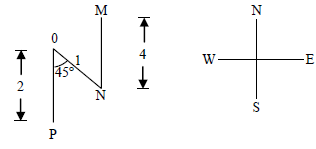# Arithmetic - Online Test

Q1. Find the sum to n terms of the series 10 + 84 + 734 + …
Explaination / Solution:

Using the answer options, substitute n = 2. The sum should add up to 94

Q2. The set of values of p for which the roots of the equation 3x2 + 2x p(p-1) = 0 are of opposite sign is
Explaination / Solution:
No Explaination.

Q3. What is the chance that a leap year, selected at random, will contain 53 Sundays?
Explaination / Solution:

There are 52 complete weeks in a calendar year = 852 × 7 =364 days Number of days in a leap year = 366 Probability of 53 Saturdays =2/7

Q4. What is the average of all multiples of 10 from 2 to 198?
Explaination / Solution: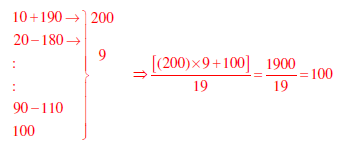Q5. The value of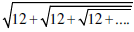is
Explaination / Solution: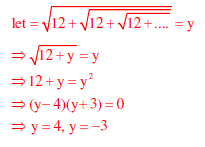Q6. If x is real and |x2 - 2x + 3| = 11 then possible values of |-x3 + x2 - x| include
Explaination / Solution: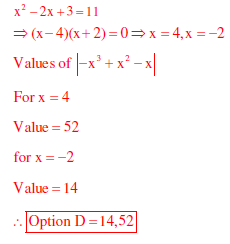Q7. At what time between 6 a.m. and 7 a.m will the minute hand and hour hand of a clock make an angle closest to 60°?
Explaination / Solution: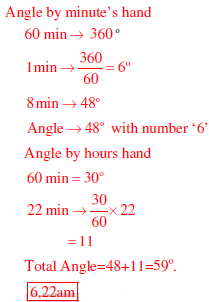Q8. Based on the given statements, select the most appropriate option to solve the given question. What will be the total weight of 10 poles each of same weight? Statements: (I) One fourth of the weight of a pole is 5 kg (II) The total weight of these poles is 160 kg more than the total weight of two poles.
Explaination / Solution:
No Explaination.

Q9. In a triangle PQR, PS is the angle bisector ofWhat is the length of PS?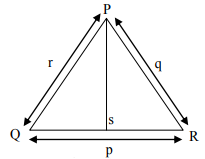Explaination / Solution:
No Explaination.

Q10. Four branches of a company are located at M,N,O, and P. M is north of N at a distance of 4 km; P is south of O at a distance of 2 km; N is southeast of O by 1 km. What is the distance between M and P in km?
Explaination / Solution: